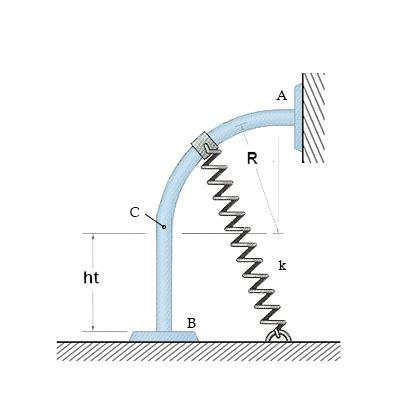# The 8 lb collar is released from rest at A and travels along the smooth guide. Determine the...

## Question:

The 8 lb collar is released from rest at {eq}A {/eq} and travels along the smooth guide. Determine the speed of the collar just before it strikes the stop at {eq}B {/eq}. The spring constant is 7 lb/in and has an unstretched length of 9 inches. {eq}R {/eq} = 13 inches and {eq}h_t {/eq} = 9 inches.## Resilience:

It is the property of a material generally spring to absorb energy when deformed under the action of applied load e.g. shock absorber. Here deformation under consideration is elastic one.

## Answer and Explanation: 1

Become a Study.com member to unlock this answer! Create your account

Unstretched length of spring = 9 in .

Stiffness of spring , k = 7 lb/in

Mass of collar = 8 lb

Unstretched spring length at A = 9 + 13 - 9 = 13

U...

See full answer below.

#### Learn more about this topic:Practice Applying Spring Constant Formulas

from

Chapter 17 / Lesson 11
3.4K

In this lesson, you'll have the chance to practice using the spring constant formula. The lesson includes four problems of medium difficulty involving a variety of real-life applications.